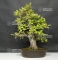##### The Japanese Bonsai specialist
Direct order Contact Help / Services Newsletter# Pseudo cydonia sinensis ref:18060182

Pseudo cydonia sinensisref. : 9943

1.900,00

voluminous/heavy item extra shipping of 12,00

Available quantity : 1Order

###### Description

Height of the tree without its pot : 760 mm. Width of the branches : 620 mm.

Size of the japanese non-enamelled pottery : 460 x 360 x 115 mm. Moyogi style.

Bonsaï coming from layering, no wire marks, grafting marks or very strong cutting wounds.

Copper-coloured foliage in spring becoming green during summer and bright orange-toned yellow in autumn.

The bark loses its scales letting us see a superb trunk. Pin-toned white flowering on spring and yellow fructification at the end of summer.

Large well-conical trunk, formed ramifications, beauty of the nebari, formed bark and flexibility of its trunk’s movement : elements that make this tree a quality subject.

Trunk’s diameter : about 110 mm. Width of the nebari : about 200 mm.

Subject coming from master Takahashi’s nursery.

Cut in may 2018.

Photographed in june 2018.

#trunk 4.5 #bonsai 3.6 #sinensis 2.8 #subject 2.7 #outdoor 2.7 #cydonia 2.7 #spring 2.6 #nebari 2.6 #pseudo 2.6 #yellow 2.6

Formule
(( ROUND((CHAR_LENGTH(b.article_nom)-CHAR_LENGTH(REPLACE(b.article_nom, 'trunk', '')))/LENGTH('trunk')) + ROUND((CHAR_LENGTH(b.article_description)-CHAR_LENGTH(REPLACE(b.article_description, 'trunk', '')))/LENGTH('trunk')) ) * 4.5) + (( ROUND((CHAR_LENGTH(b.article_nom)-CHAR_LENGTH(REPLACE(b.article_nom, 'sinensis', '')))/LENGTH('sinensis')) + ROUND((CHAR_LENGTH(b.article_description)-CHAR_LENGTH(REPLACE(b.article_description, 'sinensis', '')))/LENGTH('sinensis')) ) * 2.8) + (( ROUND((CHAR_LENGTH(b.article_nom)-CHAR_LENGTH(REPLACE(b.article_nom, 'cydonia', '')))/LENGTH('cydonia')) + ROUND((CHAR_LENGTH(b.article_description)-CHAR_LENGTH(REPLACE(b.article_description, 'cydonia', '')))/LENGTH('cydonia')) ) * 2.7) + (( ROUND((CHAR_LENGTH(b.article_nom)-CHAR_LENGTH(REPLACE(b.article_nom, 'subject', '')))/LENGTH('subject')) + ROUND((CHAR_LENGTH(b.article_description)-CHAR_LENGTH(REPLACE(b.article_description, 'subject', '')))/LENGTH('subject')) ) * 2.7) + (( ROUND((CHAR_LENGTH(b.article_nom)-CHAR_LENGTH(REPLACE(b.article_nom, 'yellow', '')))/LENGTH('yellow')) + ROUND((CHAR_LENGTH(b.article_description)-CHAR_LENGTH(REPLACE(b.article_description, 'yellow', '')))/LENGTH('yellow')) ) * 2.6) + (( ROUND((CHAR_LENGTH(b.article_nom)-CHAR_LENGTH(REPLACE(b.article_nom, 'summer', '')))/LENGTH('summer')) + ROUND((CHAR_LENGTH(b.article_description)-CHAR_LENGTH(REPLACE(b.article_description, 'summer', '')))/LENGTH('summer')) ) * 2.6) + (( ROUND((CHAR_LENGTH(b.article_nom)-CHAR_LENGTH(REPLACE(b.article_nom, 'spring', '')))/LENGTH('spring')) + ROUND((CHAR_LENGTH(b.article_description)-CHAR_LENGTH(REPLACE(b.article_description, 'spring', '')))/LENGTH('spring')) ) * 2.6) + (( ROUND((CHAR_LENGTH(b.article_nom)-CHAR_LENGTH(REPLACE(b.article_nom, 'coming', '')))/LENGTH('coming')) + ROUND((CHAR_LENGTH(b.article_description)-CHAR_LENGTH(REPLACE(b.article_description, 'coming', '')))/LENGTH('coming')) ) * 2.6) + (( ROUND((CHAR_LENGTH(b.article_nom)-CHAR_LENGTH(REPLACE(b.article_nom, 'pseudo', '')))/LENGTH('pseudo')) + ROUND((CHAR_LENGTH(b.article_description)-CHAR_LENGTH(REPLACE(b.article_description, 'pseudo', '')))/LENGTH('pseudo')) ) * 2.6) + (( ROUND((CHAR_LENGTH(b.article_nom)-CHAR_LENGTH(REPLACE(b.article_nom, 'nebari', '')))/LENGTH('nebari')) + ROUND((CHAR_LENGTH(b.article_description)-CHAR_LENGTH(REPLACE(b.article_description, 'nebari', '')))/LENGTH('nebari')) ) * 2.6)

## Secure payment## Delivery

Our logistic partners :04 74 55 23 48
Pépinière MAILLOT-BONSAÏ
Le Bois Frazy
01990 RELEVANT - FRANCE
on appointmentThe Japanese Bonsai specialist
If you like Maillot Bonsaï, share on  facebook

A vos côtés. Les services restent les mêmes.

La vente continue et les expéditions également.

Meilleurs vœux pour 2021.

Guy Maillot

OK